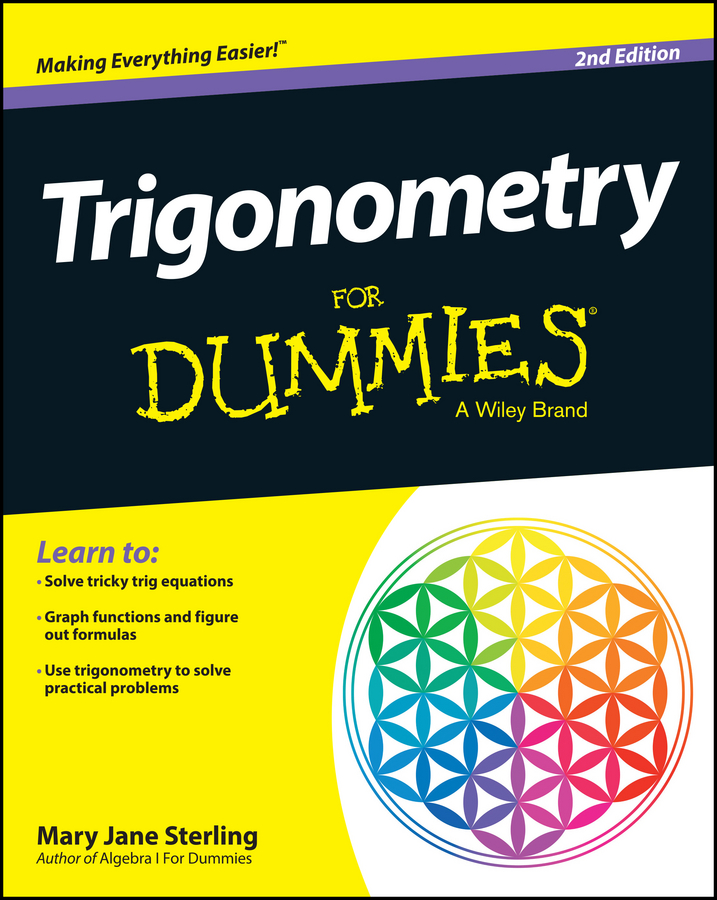##### Trigonometry For DummiesThe law of cosines comes in handy when you have two or more sides of a triangle — as in situations involving SSS and SAS — and need to use trigonometry to find the measures of the other three parts. When you have two sides, you need the angle between them. If the angle isn’t between the two sides, then you have the ambiguous case, SSA. Although such a situation isn’t impossible, you must deal with it carefully.

The law of cosines has three different versions that you can use depending on which parts of the triangle you have measures for. Notice the pattern: The squares of the three sides appear in the equations, along with the cosine of the angle opposite one of the sides — the side set equal to the rest of the stuff.

The law of cosines for triangle ABC with sides a, b, and c opposite those angles, respectively, says

a2 = b2 + c2 – 2bc cosA

b2 = a2 + c2 – 2ac cosB

c2 = a2 + b2 – 2ab cosC

In plain English, these equations say that the square of one side is equal to the squares of the other two sides, added together, minus twice the product of those two sides times the cosine of the angle opposite the side you’re solving for. Whew!